Courses

# JFET As A VVR Or VDR Electrical Engineering (EE) Notes | EduRev

## Electrical Engineering (EE) : JFET As A VVR Or VDR Electrical Engineering (EE) Notes | EduRev

The document JFET As A VVR Or VDR Electrical Engineering (EE) Notes | EduRev is a part of the Electrical Engineering (EE) Course Electronic Devices.
All you need of Electrical Engineering (EE) at this link: Electrical Engineering (EE)

JFET AS A VVR OR VDR:-

Let us consider the drain characteristics of FET as shown in the fig.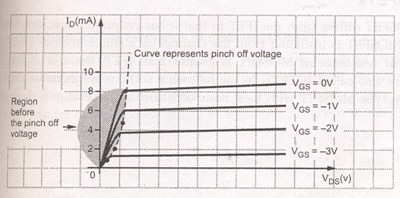In this characteristics we can see that in the region before pinch off voltage, drain characteristics are linear, i.e. FET operation is linear. In this region the FET is useful as a voltage controlled resistor, i.e. the drain to source resistance is controlled by the bias voltage VGS.( In this region only FET behaves like an ordinary resistor. This resistances can be varied by VGS). The operation of FET in the region is useful in most linear applications of FET. In such an application, the FET is also referred to as a voltage variable resistor (VVR) or voltage dependent resistor (VDR).

The drain to source conductance ( rd )

gd=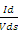for small values of Vds which may also be expressed as

gd=gd0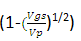where gd0 is the value of drain conductance.

When  the variation of the rwith VGS can be closely approximated by the expression

rd=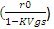Where ro = drain resistance at zero gate bias. K = a constant, dependent upon FET type.

APPLICATION OF VVR:

The VVR property of FET can be used to vary the voltage gain of a multistage amplifier A, as the signal level is increased. This action is called AGC automatic gain control. A typical arrangement is shown in the fig.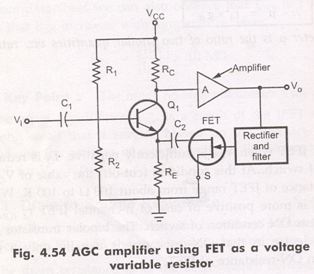Here maximum value of signal is taken rectified; filter to produce a DC voltage proportional to the output signal level. This voltage is applied to the gate of JFET, thus causing the resistance between drain and source to change. As this resistance is connected across RE, so effective RE also changes according to change in the drain to source resistance. When output signal level increases, the drain to source resistance rd increases, increasing effective RE. Increase in RE causes the gain of transistor Q1 to decrease, reducing the output signal. Exactly reverse process takes place when output signal level is decreased.

:: The output signal level is maintained constant. It is to be noted that the DC bias conditions of Q1 are not affected by JFET since FET is isolated from Q1 by capacitor C2.

Offer running on EduRev: Apply code STAYHOME200 to get INR 200 off on our premium plan EduRev Infinity!

,

,

,

,

,

,

,

,

,

,

,

,

,

,

,

,

,

,

,

,

,

;